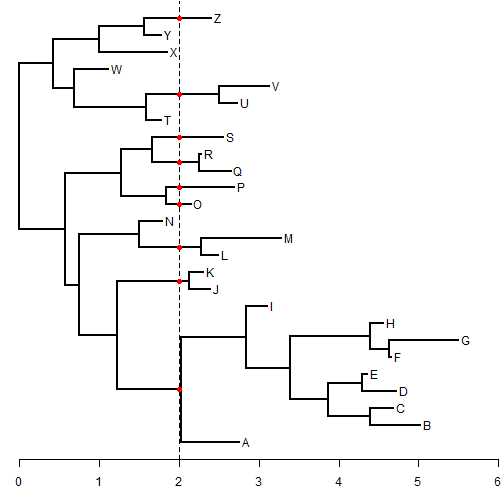## Tuesday, August 18, 2015

### Clarification & update to the phytools function treeSlice

Just to clarify how the function `treeSlice`, which bisects the tree at a particular height above the root and returns all (non-trivial or trivial & non-trivial) subtrees, is supposed to work, I thought I'd give the following quick example:

``````library(phytools)
set.seed(630)
tree<-rtree(n=26)
tree\$tip.label<-LETTERS
plotTree(tree,mar=c(3.1,rep(0.1,3)))
axis(1)
slice<-2
## mark the places where the tree should be sliced
lines(rep(slice,2),par()\$usr[3:4],lty="dashed")
obj<-get("last_plot.phylo",envir=.PlotPhyloEnv)
X<-cbind(obj\$xx[obj\$edge[,1]],obj\$xx[obj\$edge[,2]])
y<-obj\$yy[obj\$edge[,2]]
for(i in 1:nrow(X)) if(X[i,1]<slice&&X[i,2]>slice)
points(slice,y[i],pch=19,col="red")
``````From this we can see that the tree has 9 subtrees, and these subtrees should containg 9, 2, 2, 1, 1, 2, 1, 2, and 1 taxa respectively. The important point to note is that because the tree is not ultrametric, some of the taxa in the original tree are left out of the extracted subtrees.

``````subtrees<-treeSlice(tree,slice,trivial=TRUE)
print(subtrees,details=TRUE)
``````
``````## 9 phylogenetic trees
## tree 1 : 9 tips
## tree 2 : 2 tips
## tree 3 : 2 tips
## tree 4 : 1 tips
## tree 5 : 1 tips
## tree 6 : 2 tips
## tree 7 : 1 tips
## tree 8 : 2 tips
## tree 9 : 1 tips
``````
``````## tip labels
null<-lapply(subtrees,function(x) print(x\$tip.label))
``````
``````##  "A" "B" "C" "D" "E" "F" "G" "H" "I"
##  "J" "K"
##  "L" "M"
##  "O"
##  "P"
##  "Q" "R"
##  "S"
##  "U" "V"
##  "Z"
``````

For fun, we could try to make a version of `treeSlice` in which the height of the cut is (optionally) supplied interactively by the user. Here is what that might look like:

``````treeSlice<-function(tree,slice=NULL,trivial=FALSE,prompt=FALSE,...){
if(!inherits(tree,"phylo")) stop("tree should be an object of class \"phylo\".")
if(prompt){
plotTree(tree,mar=c(3.1,rep(0.1,3)),...)
axis(1)
cat("Click at the tree height where cutting is desired...\n")
flush.console()
xy<-unlist(locator(1))
slice<-xy
cat(paste("Slice height is ",signif(slice,6),". Thank you!\n",sep=""))
flush.console()
lines(rep(slice,2),par()\$usr[3:4],lty="dashed")
obj<-get("last_plot.phylo",envir=.PlotPhyloEnv)
X<-cbind(obj\$xx[obj\$edge[,1]],obj\$xx[obj\$edge[,2]])
y<-obj\$yy[obj\$edge[,2]]
if(trivial){
for(i in 1:nrow(X)) if(X[i,1]<slice&&X[i,2]>slice)
points(slice,y[i],pch=19)
} else {
for(i in 1:nrow(X))
if(X[i,1]<slice&&X[i,2]>slice&&obj\$edge[i,2]>Ntip(tree))
points(slice,y[i],pch=19)
}
}
H<-nodeHeights(tree)
edges<-which(H[,2]>slice&H[,1]<slice)
nodes<-tree\$edge[edges,2]
if(!trivial) nodes<-nodes[nodes>length(tree\$tip)]
trees<-list()
class(trees)<-"multiPhylo"
for(i in 1:length(nodes)){
if(nodes[i]>Ntip(tree)){
trees[[i]]\$root.edge<-H[which(tree\$edge[,2]==nodes[i]),2]-slice
} else {
z<-list(edge=matrix(c(2,1),1,2),
edge.length=H[which(tree\$edge[,2]==nodes[i]),2]-slice,
tip.label=tree\$tip.label[nodes[i]],Nnode=1L)
class(z)<-"phylo"
trees[[i]]<-z
}
}
return(trees)
}
``````

Try it!